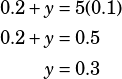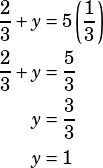1/2 x = a

x + y = 5a

In the system of equations above, a is a constant such that 0 <a <1/3. If (x,y) is a solution to the system of equations, what is one possible value of y?

(Answer: 0 < x < 1)

The easiest way to go here is to plug in! Let’s say a = 0.1, which is certainly between 0 and 1/3.

The first equation tells us:From there, we can solve for y in the second equation:So there you go—grid in 0.3 as a possible value of y and move along.

To see the whole range of possible answers, plug in the endpoints you’re given for a.

If a = 0, then the first equation tells us that x = 0, too.

If x and a both equal zero, then the second equation becomes 0 + y = 5(0), which of course means y = 0, too!

OK, now plug in 1/3 for a. The first equation tells us:Easy enough to solve for y in the second equation:So if a = 0, y = 0, and if a = 1/3, y = 1. That’s why the full range of possible answers is 0 < y < 1.